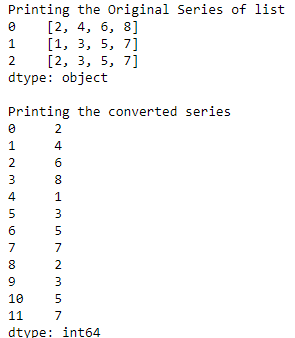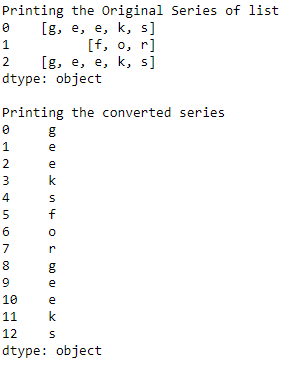# Convert Series of lists to one Series in Pandas

• Last Updated : 18 Aug, 2020

In this program, we will see how to convert a series of lists of into one series, in other words, we are just merging the different lists into one single list, in Pandas. We will be using the stack() method to perform this task. The line will be `Series.apply(Pandas.Series).stack().reset_index(drop = True)`. The reset_index() method will reset the index of the DataFrame/Series.

Example 1 : Merging multiple series lists of integers.

 `# importing the module``import` `pandas as pd`` ` `# creating a Pandas series of lists``s ``=` `pd.Series([[``2``, ``4``, ``6``, ``8``],``               ``[``1``, ``3``, ``5``, ``7``],``               ``[``2``, ``3``, ``5``, ``7``]])``print``(``"Printing the Original Series of list"``)``print``(s)`` ` `# converting series of list into one series``s ``=` `s.``apply``(pd.Series).stack().reset_index(drop ``=` `True``)`` ` `print``(``"\nPrinting the converted series"``)``print``(s)`

Output :Example 2 : Merging multiple series lists of characters.

 `# importing the module``import` `pandas as pd`` ` `# creating a Pandas series of lists``s ``=` `pd.Series([[``'g'``, ``'e'``, ``'e'``, ``'k'``, ``'s'``],``               ``[``'f'``, ``'o'``, ``'r'``],``               ``[``'g'``, ``'e'``, ``'e'``, ``'k'``, ``'s'``]])``print``(``"Printing the Original Series of list"``)``print``(s)`` ` `# converting series of list into one series``s ``=` `s.``apply``(pd.Series).stack().reset_index(drop ``=` `True``)`` ` `print``(``"\nPrinting the converted series"``)``print``(s)`

Output :My Personal Notes arrow_drop_up